## Computer Architecture Simulation & Visualisation## Atlas

The first Ferranti Atlas computer was inaugurated at the University of Manchester in December 1962. Designed by Professor Tom Kilburn and a joint University/Ferranti team, it incorporated a number of novel features, the most influential of which was Virtual Memory.

This website describes the design of the Atlas computer and explains how the HASE simulation model works. There are three versions of the model. Version 1 contains a program in its memory that demonstrates the operation of all the Atlas instructions implemented in the model. Version 2 contains a matrix multiplication program, designed to show in particular the operation of the scalar (dot) product calculation that forms the inner loop of matrix multiplication. Most early supercomputers were designed specifically to perform well on this problem, e.g. especially the Cray 1 of 1976. These two versions of the model are otherwise identical. Version 3 contains a program that computes values of a,b,c such that a² + ² = c² and a,b,c < k.

The HASE user interface contains three windows. Figure 1 shows an image of the HASE user interface with the simulation model of Atlas in the main (right hand) Project View pane, model parameters (e.g. register and store contents) in the (left hand) Project Inspector pane. The lower, Output pane shows information produced by HASE. The icons in the top row allow the user to load a model, compile it, run the simulation code thus created and to load the trace file produced by running a simulation back into the model for animation.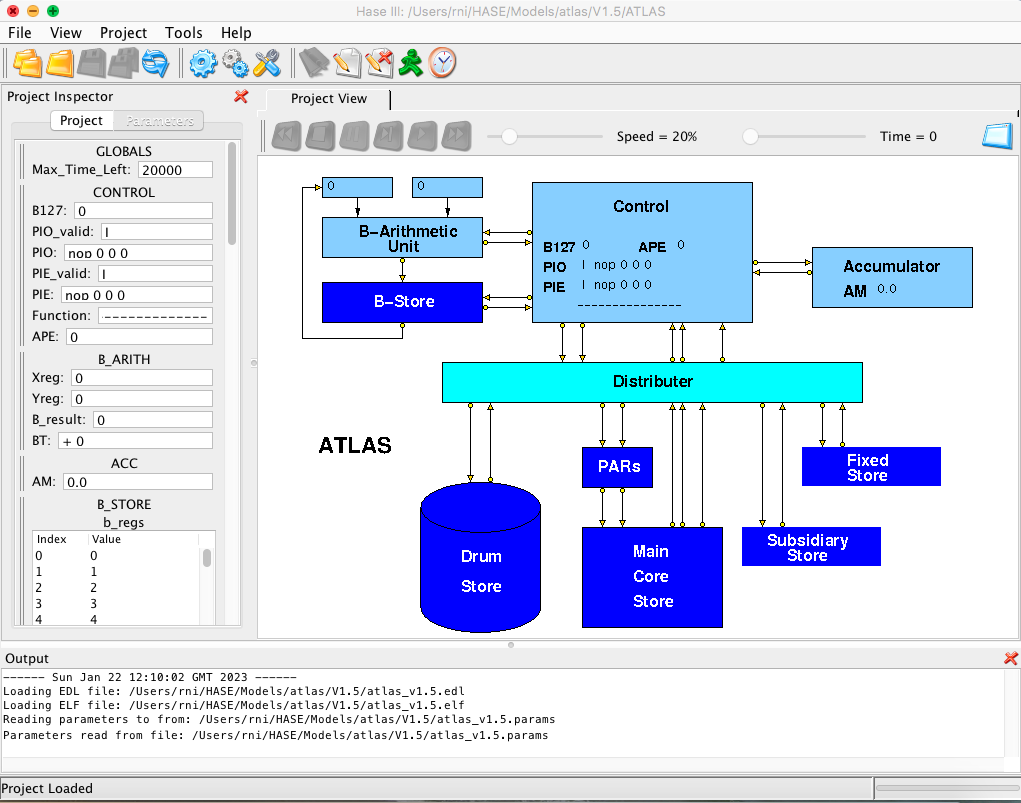Figure 1. The Atlas simulation model loaded into HASE

Once a trace file has been loaded, the animation control icons at the top of the Project View window become active (Figure 2). From left to right, these allow the animation to be rewound, stopped, paused, single stepped, run or fast forwarded to the end. As the animation proceeds, packets of information can be seen passing between entities while the entities themselves change colour to reflect their states (idle, busy, waiting).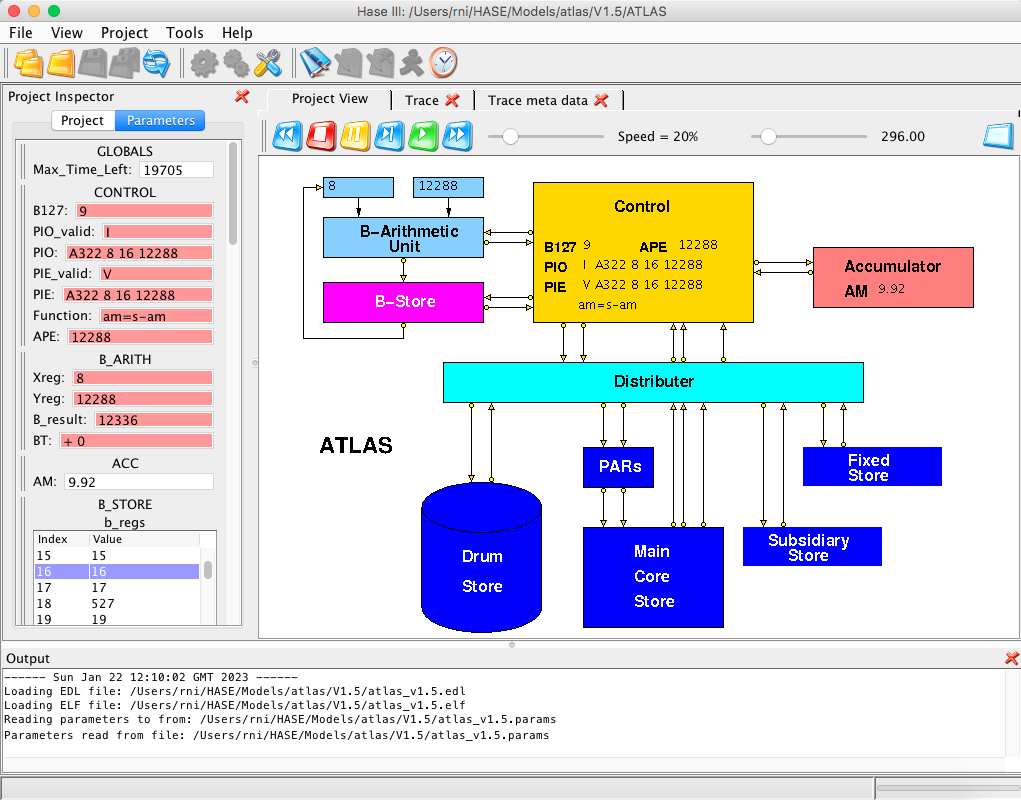Figure 2. The Atlas simulation model during animation

The model contains 10 entities: the B-Arithmetic Unit, the B-Store, the Control unit, the Accumulator, the Distributer, the Page Address Registers (PARs) and the Drum, Core, Subsidiary and Fixed Stores. In Figure 2 the B-Store and the Accumulator are busy, the Control unit is waiting and the rest are idle.

## The Atlas instruction set

Atlas had a 48-bit instruction word made up of a 10-bit function code, two 7-bit index addresses (Ba and Bm) and a 24-bit store address (Figure 3). Functions that operated on the Accumulator (A-codes) were thus one-address with double B-modification. B-line 0 always returned a value of 0, thus allowing for singly modified or unmodified accesses. The most significant bit of the address field distinguished between user addresses and system addresses, while the three least significant bits were used to address one of eight 6-bit characters within a store word.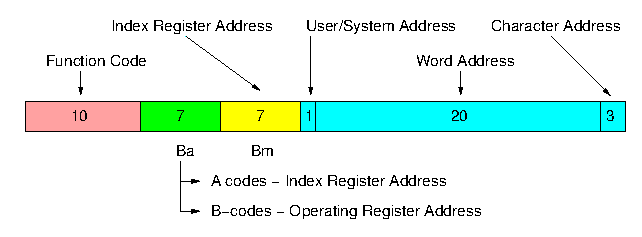Figure 3. Atlas instruction format

The B-codes involved operations on one of the 128 B-lines specified by the Ba field in the instruction, Bm being used to specify a single modifier. The B-store was implemented as a fast core store (0.7 microsecs cycle time) of 120 24-bit words, together with eight flip-flop registers which served special purposes. These included the floating-point accumulator exponent, for example, and the three control registers (or program counters) used for user programs, extracode and interrupt control. The existence of these three control registers allowed the machine to switch rapidly from one context to another, while their inclusion within the addressable B-store avoided the need for separate control transfer functions. Extracodes were a set of functions which gave access to some 250 built-in subroutines held in the high-speed (0.5 microsecs) read-only fixed store. These included the more complex mathematical functions such as sin, log, etc., peripheral control orders, input/output conversion routines, etc. The extracodes made use of 30 of the B-lines, leaving 90 for use as index registers or fixed-point accumulators in user programs.

User addresses in Atlas (defined by a 0 in their most significant address bit) referred to a 1M word virtual address space, and were translated into real addresses through a set of page registers. The real store consisted of a total of 112K words of core and drum storage, combined together through the paging mechanism so as to appear to the user as a one-level store . Thus a user’s total working store requirements could not exceed this amount, but code and data could be placed in any convenient part of the virtual address space. This led to an informal segmentation of the address space, a concept developed formally at MIT in MULTICS and at Manchester in MU5, the successor to Atlas.

Atlas addresses with a 1 in the most significant bit were system addresses rather than user virtual addresses, and referred to the fixed store (containing the extracodes), the subsidiary store (used as working space by the extracodes and operating system), or the V-store. The latter contained the various registers needed to control the tape decks, input/output devices, paging mechanism, etc., thus avoiding the need for special functions for this purpose. This technique of incorporating peripherals into the address space was subsequently used in a number of computer systems, notably the DEC PDP-11, and is now in common use as 'memory-mapped I/O'.

## The Simulation Model

In any simulation modelling system there is a trade-off between accuracy and performance. HASE was designed primarily as a high-level visualisation tool for computer architecture students and therefore simulates systems at register/word level rather than bit level. This inevitably imposes some limitations on the way models can be constructed, e.g. registers are modelled using typed variables and stores are modelled as arrays of typed variables. Thus the HASE Atlas simulation model is intended to provide a visual demonstration of the workings of the processor and memory system, rather than being designed to run real Atlas programs.

The three versions of the model available from the website contain different sequences of instructions. The first is designed to demonstrate the operation of each of the instructions implemented in the model, while the second demonstrates the operation of matrix multiplication, one of the most frequently used algorithms in supercomputers. The third is an adaptation of a program that finds values for the lengths of the sides of Pythagorean right triangles.

### The Drum and Core Stores

HASE models memories as arrays of elements which can be integers, floating-point numbers or instructions. Since these elements cannot be mixed together in a single array, the model takes advantage of the Atlas paging system by modelling the Drum Store as a set of pages and the Core Store as a set of blocks, each made up of 512 words, as in Atlas (though only 256 words are instantiated in the model for performace reasons), but with different blocks/pages containing different types of element.

At the start of each simulation the program and its data are contained in the Drum Store while the Core Store is empty (i.e. contains zeroes). The program code is in page 0 of the Drum, fixed-point integers are in page 2 and floating-point reals in page 3. In the Core Store Block 0 is modelled as an instruction array, Block 1 as an integer array and Block 2 as a floating-point array. These arrays are themselves loaded from files such as DRUM_STORE.page0.mem when the simulation model is loaded into HASE.

The Fixed Store and Subsidiary Store are also included as entities in the model. The Subsidiary Store is used only in the third of the example programs while the Fixed Store is not used at all.

The PARs are modelled as a set of registers, each of which contains (from left to right in the Project Inspector pane) a use bit, a valid bit and an address. The initiallsation file for the Page Address Registers (PARs) sets all the PARs to zero (and thus invalid) at the start of a simulation. The first action in the simulation is an instruction access to word 0 of the virtual memory (because B127, the Program Counter, is initially set to 0). This causes a page fault in the PARs. The PARs are coded to imitate the actions of the Atlas Supervisor (the Atlas operating system) by copying page 0 from the Drum Store to block 0 of the Core Store and setting the first PAR to point to it. The actual movement of data is not shown in the visualistion (it would be very tedious to watch) but the Core Store contents are shown being updated when the first store request reaches it.

Subsequently, when accesses are made for data, similar actions occur on the first access. The first access to a fixed-point value causes a page transfer between block 2 of the Drum and page 1 of the Core, the first floating-point access a transfer between block 3 of the Drum and page 2 of the Core. A full demonstration of the paging system is beyond the scope of the current model.

### Instruction Format

Instructions are modelled as closely as possible to the Atlas format, with the Ba, Bm and Address fields being modelled as integers. However, HASE models the function field of instructions as elements of an enumerated type and these elements cannot be integers. Functions in the model are therefore of the form A314, B121, etc, which actually aids legibility.

### Floating-point Arithmetic

The model implements floating-point arithmetic using the floating-point operations provided by the underlying hardware of the computer on which the simulation runs, accessed via standard C++ operations. This is not an accurate representation of the way Atlas performed these operations, of course, but is adequate for the purposes of this demonstration. To simulate the 48-bit floating-point arithmetic used in Atlas would require bit-level coding of the Accumulator unit and would not be especially instructive, except to floating-point arithmetic gurus.

For the same reasons, the model does not implement the full set of Atlas floationg-point instructions, nor does it attempt to model the Accumulator as a double-length register. It just implements simple load, store, add, subtract, multiply and divide operations on AM, the most significant half of the Atlas double-length accumulator. Also, B124, the floating-point exponent register in Atlas, is not implemented.

## Demonstration Program

Version 1 of the model contains the demonstration program shown in Table 1 (below), together with some appropriate data. The program is initially held in Page 0 of the Drum Store and is copied into Block 0 of the Core Store following the page fault caused by the first instruction access. The integers held initially in Page 2 of the Drum Store are copied into Block 1 of the Core Store when the first integer operand access causes a page fault and likewise the floating-point numbers initially held in Page 3 of the Drum Store are copied into Block 2 of the Core Store. The program is essentially a test program that executes each of the instructions implemented in the model. These include all the B functions and the A functions as described above. The table shows the actions performed for each instruction and the resulting outcome.

AM = Accumulator, VS = Virtual Store, CS B2W8 = Core Store Block 2, Word 8

Table 1. The demonstration program

## Matrix Multiplication Program

The matrix multiplication program contained in Version 2 of the HASE Atlas simulation model multiplies together a 4x3 matrix X and a 3x4 matrix Y to produce a 4x4 matrix Z, as shown in Figure 4. The first element of the first row of Z is the scalar (dot) product of the first row of X and the first column of Y, the second is the scalar product of the first row of X with the second column of Y, etc.

Table 2 shows the program, which is in the form of a triple nested loop. The outer loop increments the X row at each iteration, the middle loop increments the Y column at each iteration while the inner loop forms the scalar products.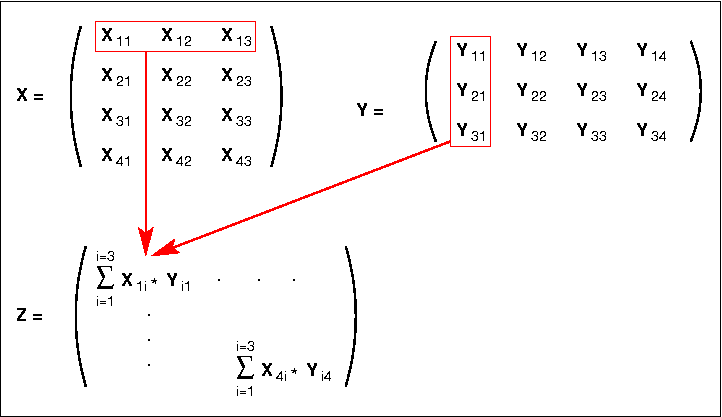Figure 4. Matrix multiplication example

 B127 Instruction Purpose/Action Result 0 B121 1 24 0 B1 = X row length / Y column length (x8) B1 = 24 1 B121 2 32 0 B2 = X column length / Y row length (x8) B2 = 32 2 B121 3 0 0 B3 = current X row number B3 = 0 3 B121 4 0 0 B4 = address of first column of current X row B4 = 0 4 B121 5 0 0 B5 = current X column number B5 = 0 5 B121 6 0 0 B6 = current Y column number B6 = 0 6 B121 7 0 0 B7 = address of Y row element B7 = 0 7 B121 8 0 0 B8 = Z index number (x8) B8 = 0 8 A314 4 5 12288 Start of Loop: Loads ACC with element of X ACC = Xji 9 A362 6 7 12384 Multiplies ACC by element of Y ACC * Yij 10 A320 8 0 12480 Adds element of Z to ACC 11 A356 8 0 12480 Writes ACC to element of Z 12 B124 5 0 8 Increment word address of X column number B5 + 8 13 B124 7 2 0 Increment word address of Y row number B7 + B2 14 B172 5 1 0 Test B5 against B1 Sets BT 15 B225 127 0 8 If BT /=0, jump back to start of Loop B127 = 8 16 B121 5 0 0 Resets X column number to 0 B5 = 0 17 B124 6 0 8 Increments Y column number B6 + 8 18 B121 7 0 0 Resets Y row number to 0 B7 = 0 19 B124 8 0 8 Increments Z index B8 + 8 20 B172 6 2 0 Test B6 against B1 Sets BT 21 B225 127 0 8 If BT /=0, jump back to start of Loop B127 = 8 22 B124 3 0 8 Increments X row number B3 + 8 23 B124 4 1 0 Increment X row address B4 + B1 24 B121 6 0 0 Resets Y column number B6 = 0 25 B172 3 2 0 Tests B3 against B2 Sets BT 26 B225 127 0 8 If BT /=0, jump back to start of Loop B127 = 8 27 STOP

Table 2. The matrix multiplication program

## Sums of Squares

Version 3 of the HASE Atlas model contains a program written by John Buckle in 1962 as a Ferranti training exercise. Figure 5 shows the original code with comments (which include one minor mistake) and the corresponding HASE Atlas simulation code. The value of k is in virtual store word 1024. The program uses three extracodes E1302, E1064 and E1067. E1302 is a B multiply instruction, implemented in the model simply as an instruction executed directly in the B Arithmetic unit. E1064 and E1067 are implemented by the Control Unit and write values into the Subsidiary Store which are printed at the end of the simulation run in the output pane of the HASE GUI. This is not how it would have worked in the real Atlas, of course, but simply a device to enable the sum of squares program to be demonstrated at the Atlas 50th Anniversary Symposium.

The value of k in the model is set at 15, so the model produces the results:

3, 4, 5
5, 12, 13
6, 8, 10
9, 12, 15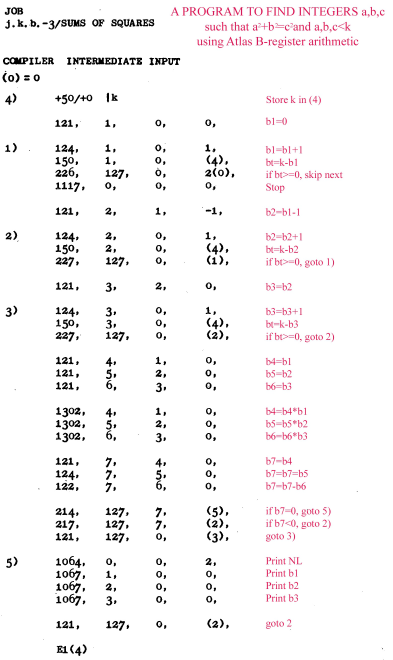B127 0 B121 8 0 0 1 B121 1 0 0 2 1) B124 1 0 1 3 B150 1 0 8192 4 B226 127 0 6 if bt>=0, skip next 5 STOP 6 B121 2 1 -1 b2=b1-1 7 2) B124 2 0 1 b2=b2+1 8 B150 2 0 8192 bt=k-b2 9 B227 127 0 2 if bt>=o, ->1) 10 B121 3 2 0 b3 = b2 11 3) B124 3 0 1 b3=b3+1 12 B150 3 0 8192 bt=k-b3 13 B227 127 0 7 if bt>=0, ->2) 14 B121 4 1 0 b4=b1 15 B121 5 2 0 b5=b2 16 B121 6 3 0 b6=b3 17 E1302 4 1 0 b4=b4*b1 18 E1302 5 2 0 b5=b5*b2 19 B1302 6 3 0 b6=b6*b3 20 B121 7 4 0 b7=b4 21 B124 7 5 0 b7=b7+b5 22 B122 7 6 0 b7=b7-b6 23 B214 127 7 26 if b7=0, ->5) 24 B217 127 7 7 if b7<0, ->2) 25 B121 127 0 11 -> 3) 26 5) E1064 0 0 0 print NL 27 E1067 1 0 0 print b1 27 E1067 2 0 0 print b2 27 E1067 3 0 0 print b3 29 B124 8 0 24 b8=b8+24 30 B121 127 0 7 -> 2)
Figure 5. Sums of Squares

## Reference

1. ^ T. Kilburn, D.B.G. Edwards, M.J. Lanigan and F.H. Sumner
"One-level Storage System"
IRE Trans., Vol EC-11, pp 223-234 1962